cancel
Showing results for
Did you mean:Anonymous
Not applicable

## Combine all possible values from a single column- Power query

Raw DataHow can I get all the possible combination of 7 companies with unique ID?

-Count 1-7

-All Combination of company, e.g. count=1, only show A/B/C/D/E/F/G price in one row

-Sum of the combined companies price

1 ACCEPTED SOLUTIONSuper User

@Anonymous Using my code I referenced previously, I turned it into a function fn_Subsets that transforms a list into a list of subsets (a list of lists).

``````(L as list) as list =>
let
N = List.Count(L),
Subsets =
List.Transform(
{0..Number.Power(2, N)-1},
(i) => List.Transform(
{0..N-1},
(j) => if Number.Mod(Number.IntegerDivide(i, Number.Power(2, j)), 2) = 1
then L{j}
else null
)
),
RemoveNulls = List.Transform(Subsets, each List.RemoveNulls(_))
in
RemoveNulls``````

We can apply this function in a Group By set to each set of companies associated with each ID.

Here's a complete sample query (including the function definition) you can paste into the Advanced Editor of a new blank query.

``````let
/*Define a list function. This is usually done in a separate query.*/
fn_Subsets = (L as list) as list =>
let
N = List.Count(L),
Subsets =
List.Transform(
{0..Number.Power(2, N)-1},
(i) => List.Transform(
{0..N-1},
(j) => if Number.Mod(Number.IntegerDivide(i, Number.Power(2, j)), 2) = 1
then L{j}
else null
)
),
RemoveNulls = List.Transform(Subsets, each List.RemoveNulls(_))
in
RemoveNulls,

/*Define sample dataset. Replace with your own data.*/
Source = Table.FromRows(Json.Document(Binary.Decompress(Binary.FromText("Rcy7DcAgFEPRXVxTBPIvSQjkMwJ6+68RIyG5uJJP41oRPRwi8zDXebAgnmwVE9vEi+1ibleDXJr7d+C+2Sg+bBJfNosfW2D2Aw==", BinaryEncoding.Base64), Compression.Deflate)), let _t = ((type nullable text) meta [Serialized.Text = true]) in type table [ID = _t, Company = _t, Price = _t]),
SampleData = Table.TransformColumnTypes(Source,{{"ID", type text}, {"Company", type text}, {"Price", Int64.Type}}),

/*Logic applying the subsets function and aggregating the results.*/
#"Grouped Rows" = Table.Group(SampleData, {"ID"}, {{"SubsetList", each fn_Subsets([Company]), type list}}),
#"Expanded Count" = Table.ExpandListColumn(#"Grouped Rows", "SubsetList"),
#"Filtered Rows" = Table.SelectRows(#"Added Custom", each ([Company] <> "")),
#"Expanded SubsetList" = Table.ExpandListColumn(#"Filtered Rows", "SubsetList"),
#"Merged Queries" = Table.NestedJoin(#"Expanded SubsetList", {"ID", "SubsetList"}, SampleData, {"ID", "Company"}, "Expanded SubsetList", JoinKind.LeftOuter),
#"Expanded Expanded SubsetList" = Table.ExpandTableColumn(#"Merged Queries", "Expanded SubsetList", {"Price"}, {"Price"}),
#"Aggregate Rows" = Table.Group(#"Expanded Expanded SubsetList", {"ID", "Company"}, {{"Sum_Price", each List.Sum([Price]), type nullable number}, {"No of Supplier", each Table.RowCount(_), Int64.Type}}),
#"Sorted Rows" = Table.Sort(#"Aggregate Rows",{{"ID", Order.Ascending}, {"No of Supplier", Order.Ascending}, {"Company", Order.Ascending}})
in
#"Sorted Rows"``````

12 REPLIES 12Helper I
1. First, you must group the table by ID and return all rows and perform the sum of the price.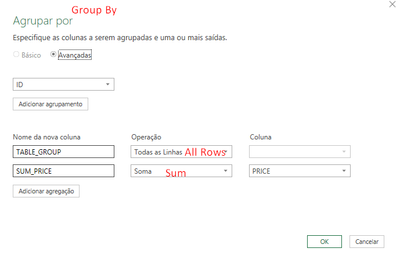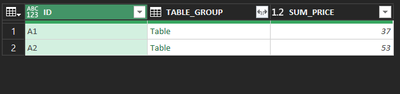2. After, add a new custom column and use the function Combiner.CombineTextByDelimiter. Use the delimiter "/" and combine the collumn from grouped table COMPANY;

``= Combiner.CombineTextByDelimiter("/")([TABLE_GROUP][COMPANY])``Finally, only select the columns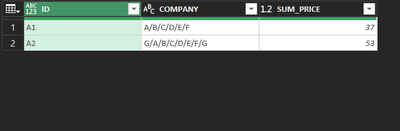Sample M

``````let
Fonte = Excel.CurrentWorkbook(){[Name="sAMPLE"]}[Content],
GroupID = Table.Group(Fonte, {"ID"}, {{"TABLE_GROUP", each _, type table [ID=nullable text, COMPANY=nullable text, PRICE=nullable number]}, {"SUM_PRICE", each List.Sum([PRICE]), type nullable number}}),
CombineCompany = Table.AddColumn(GroupID, "COMPANY", each Combiner.CombineTextByDelimiter("/")([TABLE_GROUP][COMPANY]), type text),
SelectColumns = Table.SelectColumns(CombineCompany,{"ID", "COMPANY", "SUM_PRICE"})
in
SelectColumns``````Anonymous
Not applicable

Thank you for your comment. But I would like to know how many possible combination and its sum of price for 1to 7 suppliers.

e.gSuper User

Hi @Anonymous ,

With the brilliant code provided by @AlexisOlson, I applied it to your case. This is not achievable without Alexis' code. Very smart!!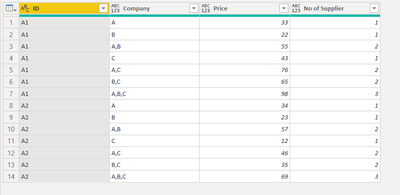Open a blank query and paste over the code below (delete out the existing code first).

Code:

let
Source = Table.FromRows(Json.Document(Binary.Decompress(Binary.FromText("i45WcjRU0lFyBGJjY6VYHSjfCYiNjBB8ZyA2gcobwdSbIPhg9UjyIPWGQP2xAA==", BinaryEncoding.Base64), Compression.Deflate)), let _t = ((type nullable text) meta [Serialized.Text = true]) in type table [ID = _t, Company = _t, Price = _t]),
#"Changed Type" = Table.TransformColumnTypes(Source,{{"ID", type text}, {"Company", type text}, {"Price", Int64.Type}}),

//Group rows to do the count of companies
#"Grouped Rows" = Table.Group(#"Changed Type", {"ID"}, {{"Count", each Table.RowCount(_), Int64.Type}}),

//Combination transformation - Company
Subset = Table.AddColumn(#"Grouped Rows", "Subset", each List.Transform(
{0..Number.Power(2, [Count])-1},
(i) => List.Transform(
{0..[Count]-1},
(j) => if Number.Mod(Number.IntegerDivide(i, Number.Power(2, j)), 2) = 1
then Table.SelectRows(#"Changed Type", (x)=>x[ID]=[ID])[Company]{j}
else null
)
)),
Concatenate = Table.AddColumn(Subset, "Concatenate", each List.Transform([Subset], each Text.Combine(List.RemoveNulls(_), ","))),

//Combination transformation - Price
#"Subset\$" = Table.AddColumn(Concatenate, "Subset \$", each List.Transform(
{0..Number.Power(2, [Count])-1},
(i) => List.Transform(
{0..[Count]-1},
(j) => if Number.Mod(Number.IntegerDivide(i, Number.Power(2, j)), 2) = 1
then Table.SelectRows(#"Changed Type", (x)=>x[ID]=[ID])[Price]{j}
else null
)
)),
#"Concatenate\$" = Table.AddColumn(#"Subset\$", "Concatenate\$", each List.Transform([#"Subset \$"], each List.Sum(List.RemoveNulls(_)))),

//Join list (Company & Price)

//Expand join list
#"Expanded Combine" = Table.ExpandListColumn(#"Added Custom", "Company"),

//Expand join sub-list
#"Expanded Combine1" = Table.ExpandListColumn(#"Expanded Combine", "Company"),

//Aligning Company and Price

//Filter out unnecessary rows
#"Filtered Rows1" = Table.SelectRows(#"Added Custom2", each ([Filter] = true)),

//Remove unnecessary columns
#"Removed Other Columns" = Table.SelectColumns(#"Filtered Rows1",{"ID", "Company", "Price" }),

//number of supplier
in

Regards

KTSuper User

Hi @Anonymous ,

Please see code below (highlighted code show is the code to get the combination):

let
Source = Table.FromRows(Json.Document(Binary.Decompress(Binary.FromText("i45WcjRU0lFyBGJjY6VYHSjfCYiNjBB8ZyA2gcobwdSbIPhg9UjyIPWGQP2xAA==", BinaryEncoding.Base64), Compression.Deflate)), let _t = ((type nullable text) meta [Serialized.Text = true]) in type table [ID = _t, Company = _t, Price = _t]),
#"Changed Type" = Table.TransformColumnTypes(Source,{{"ID", type text}, {"Company", type text}, {"Price", Int64.Type}}),
#"Extracted Values" = Table.TransformColumns(#"Added Custom", {"Count", each Text.Combine(List.Transform(_, Text.From), ";"), type text})
in
#"Extracted Values"Regards

KTAnonymous
Not applicable

Thank you for your comment. But I would like to know how many possible combination and its sum of price for 1to 7 suppliers within the same ID

e.g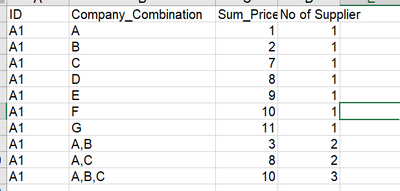Super User

You might be interested in my comment here. It has all the basic math needed.

Note that there are 2^7 = 128 combinations for 7 companies. The size can get out of hand really quickly if you try to precompute all possibilities. I'm not sure what your ultimate goal is, but it might be better not to try to precompute everything but rather calculate combinations with DAX measures.Super User

@Anonymous Using my code I referenced previously, I turned it into a function fn_Subsets that transforms a list into a list of subsets (a list of lists).

``````(L as list) as list =>
let
N = List.Count(L),
Subsets =
List.Transform(
{0..Number.Power(2, N)-1},
(i) => List.Transform(
{0..N-1},
(j) => if Number.Mod(Number.IntegerDivide(i, Number.Power(2, j)), 2) = 1
then L{j}
else null
)
),
RemoveNulls = List.Transform(Subsets, each List.RemoveNulls(_))
in
RemoveNulls``````

We can apply this function in a Group By set to each set of companies associated with each ID.

Here's a complete sample query (including the function definition) you can paste into the Advanced Editor of a new blank query.

``````let
/*Define a list function. This is usually done in a separate query.*/
fn_Subsets = (L as list) as list =>
let
N = List.Count(L),
Subsets =
List.Transform(
{0..Number.Power(2, N)-1},
(i) => List.Transform(
{0..N-1},
(j) => if Number.Mod(Number.IntegerDivide(i, Number.Power(2, j)), 2) = 1
then L{j}
else null
)
),
RemoveNulls = List.Transform(Subsets, each List.RemoveNulls(_))
in
RemoveNulls,

/*Define sample dataset. Replace with your own data.*/
Source = Table.FromRows(Json.Document(Binary.Decompress(Binary.FromText("Rcy7DcAgFEPRXVxTBPIvSQjkMwJ6+68RIyG5uJJP41oRPRwi8zDXebAgnmwVE9vEi+1ibleDXJr7d+C+2Sg+bBJfNosfW2D2Aw==", BinaryEncoding.Base64), Compression.Deflate)), let _t = ((type nullable text) meta [Serialized.Text = true]) in type table [ID = _t, Company = _t, Price = _t]),
SampleData = Table.TransformColumnTypes(Source,{{"ID", type text}, {"Company", type text}, {"Price", Int64.Type}}),

/*Logic applying the subsets function and aggregating the results.*/
#"Grouped Rows" = Table.Group(SampleData, {"ID"}, {{"SubsetList", each fn_Subsets([Company]), type list}}),
#"Expanded Count" = Table.ExpandListColumn(#"Grouped Rows", "SubsetList"),
#"Filtered Rows" = Table.SelectRows(#"Added Custom", each ([Company] <> "")),
#"Expanded SubsetList" = Table.ExpandListColumn(#"Filtered Rows", "SubsetList"),
#"Merged Queries" = Table.NestedJoin(#"Expanded SubsetList", {"ID", "SubsetList"}, SampleData, {"ID", "Company"}, "Expanded SubsetList", JoinKind.LeftOuter),
#"Expanded Expanded SubsetList" = Table.ExpandTableColumn(#"Merged Queries", "Expanded SubsetList", {"Price"}, {"Price"}),
#"Aggregate Rows" = Table.Group(#"Expanded Expanded SubsetList", {"ID", "Company"}, {{"Sum_Price", each List.Sum([Price]), type nullable number}, {"No of Supplier", each Table.RowCount(_), Int64.Type}}),
#"Sorted Rows" = Table.Sort(#"Aggregate Rows",{{"ID", Order.Ascending}, {"No of Supplier", Order.Ascending}, {"Company", Order.Ascending}})
in
#"Sorted Rows"``````Anonymous
Not applicable

Raw Data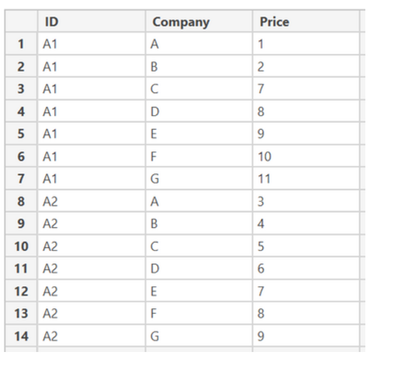Result ExampleHow to get the 7! (factorial) number of all possible combination on company col for the unique ID?

I would like to have 1 to 7  of suppliers and see the all the possible combinations.Super User

7! is the number of permutations of seven companies, not the number of possible combinations.

https://community.powerbi.com/t5/Power-Query/Combine-all-possible-values-from-a-single-column-Power-...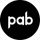Anonymous
Not applicable

Hi @miguel ,

As I havent got the corrected answer , I had created one new question for more elaboration!Super User

Hi @Anonymous ,

I'll have a think through about what can be done. It is easy to find out how many combinations but to list them out in a way like A; A B; A C ....... It is quite a challenge, but I will have a go.

Regards

KT